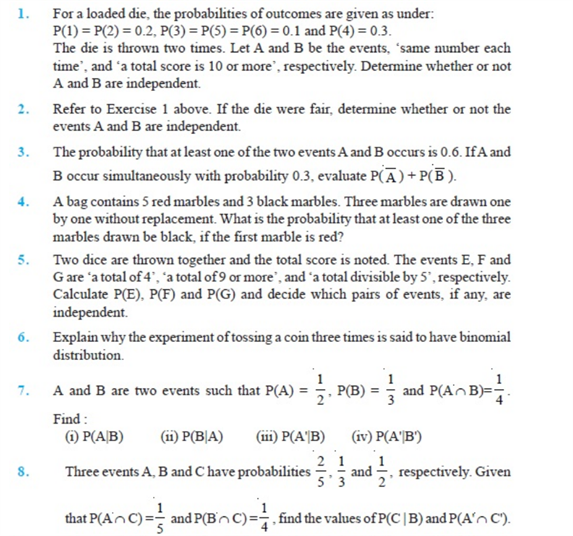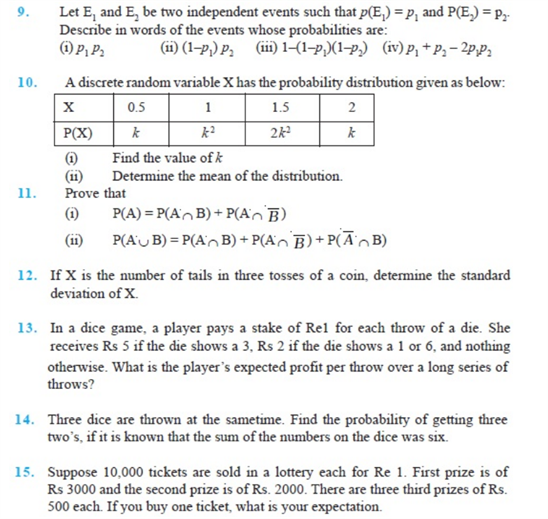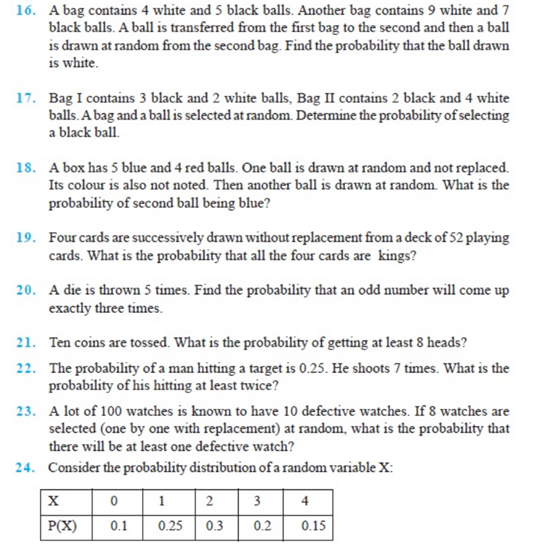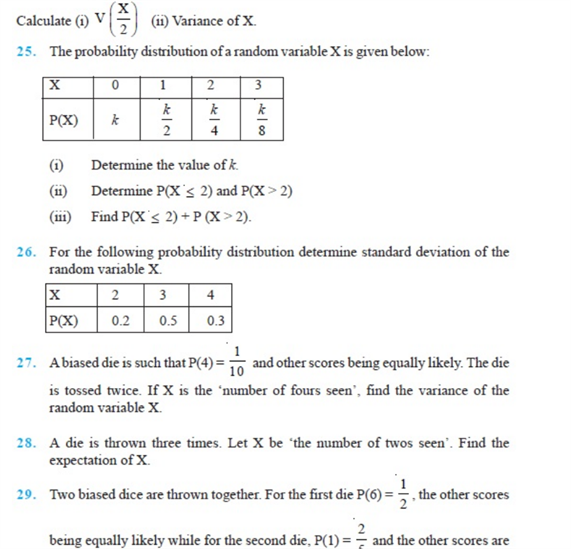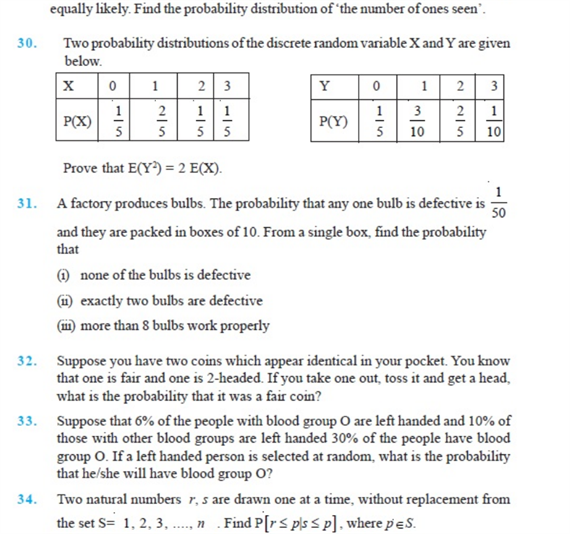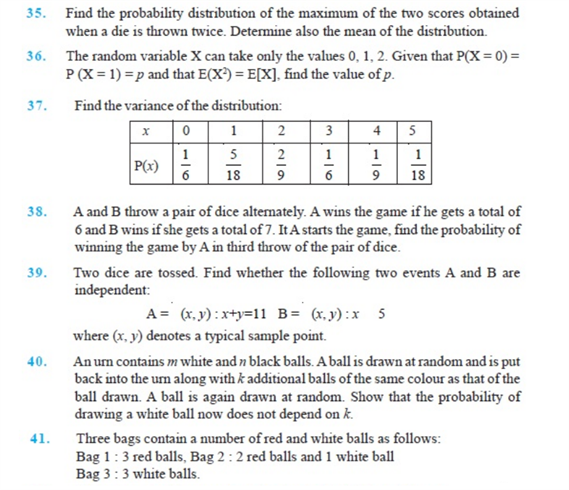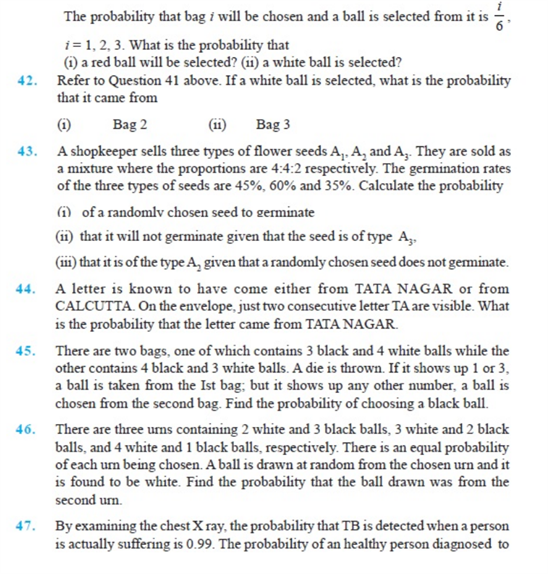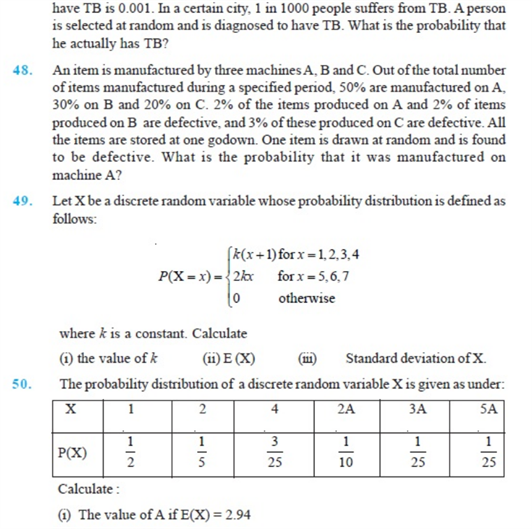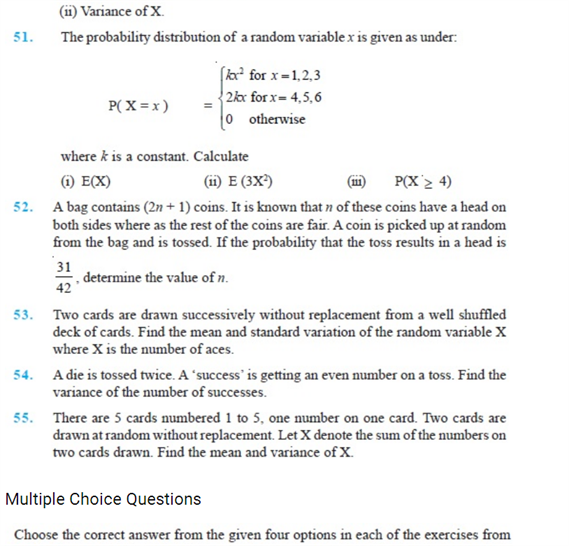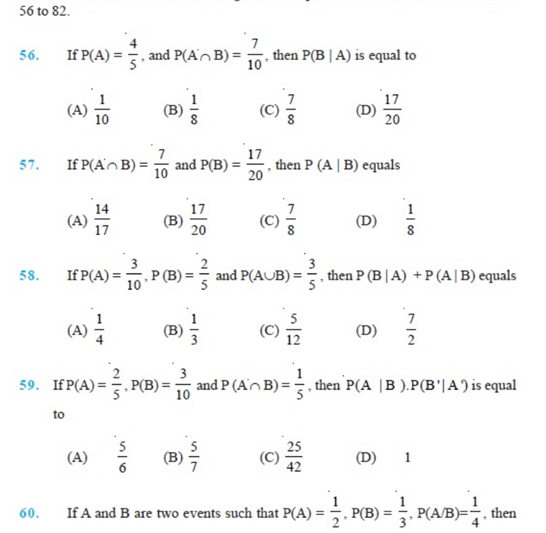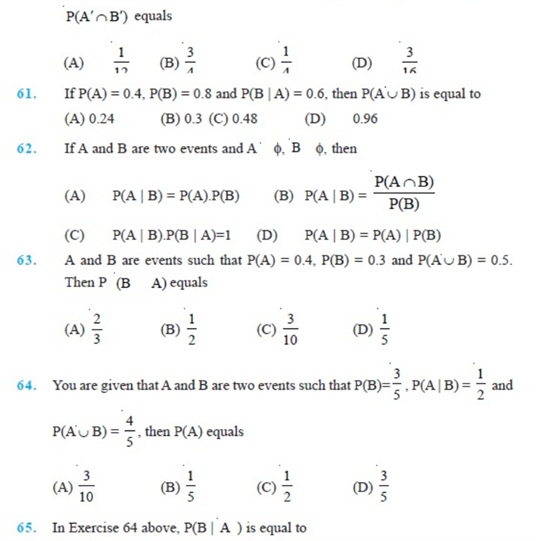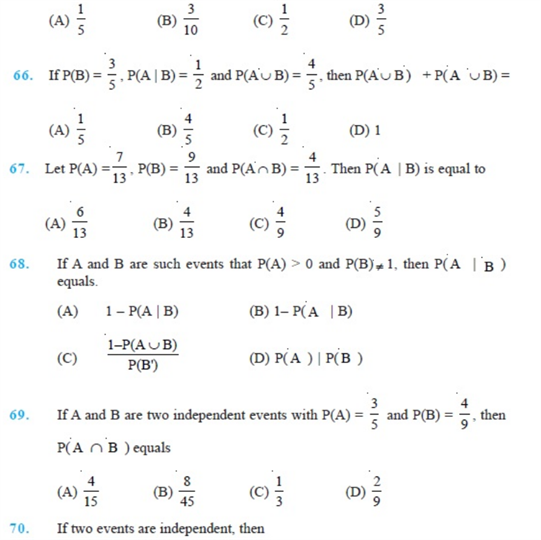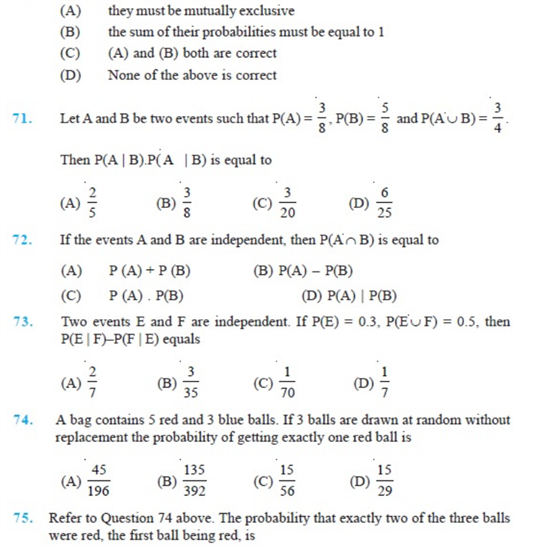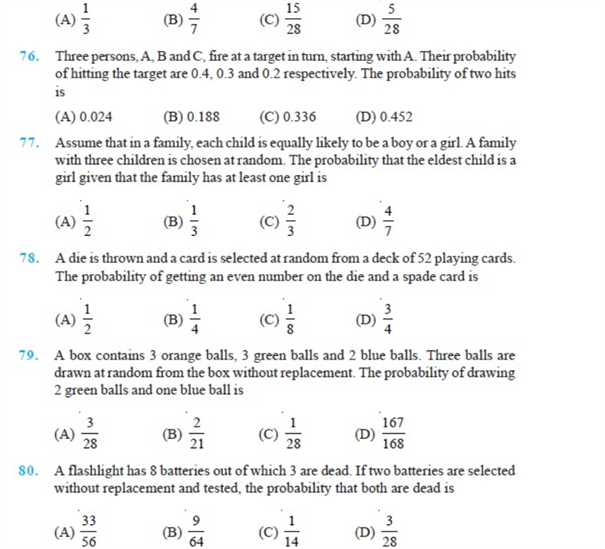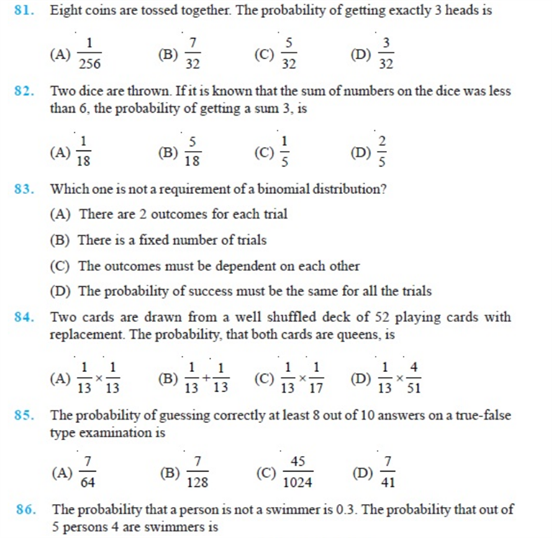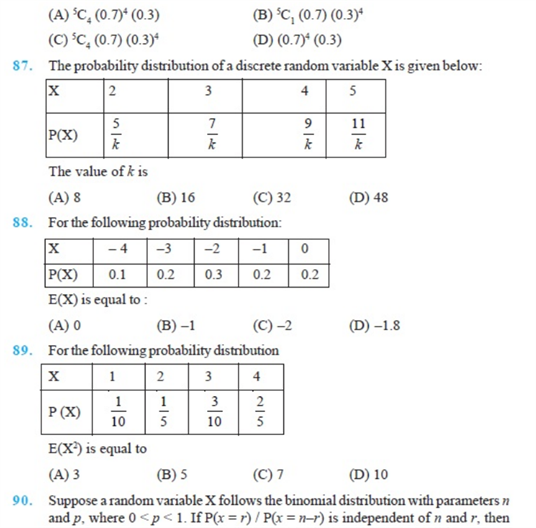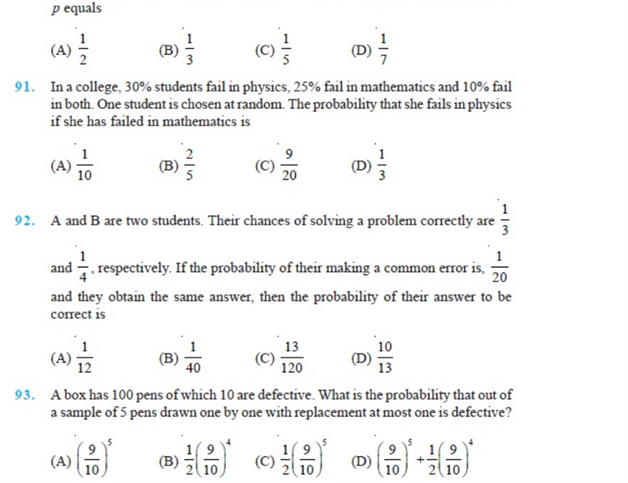# Chapter 13 - Probability

Probability Chapter 13 of Class 12 is one of the most important topics in Maths CBSE Board Exams. The important questions of class 12 chapter -13 – Probability will help you to practice well for exams. Solving these questions will help you in being confident about the topic.

After solving these important questions on You can practice the given NCERT Questions too. These questions of Chapter 13 class 12 contains all types of questions – short and long. This topic is also important for preparing for entrance exams.

Also, check:

## Important Questions Of Probability Chapter 13 – Probability

1) Two numbers are selected at random from the integers 1 through 9. If the sum is even, find the probability that both the numbers are odd. (Answer: 5/8).

2)A die is rolled. If the outcome is an odd number, what is the probability that it is prime? (Answer: 2/3).

3)A coin is tossed twice. If the outcome is at most one tail, what is the probability that both head and tail have appeared? (Answer: 2/3).

4)An unbiased die is tossed twice. Find the probability of getting a 4, 5, 6on the first toss and a 1, 2, 3, 4 on the second toss. (Answer: 1/3).

5)The probability that A hits a target is 1/3 and the probability that B hits it is 2/5. What is the probability that the target will be hit if both A and B shoot at it? (Answer: 3/5).

### NCERT Questions Chapter-13 Class 12 (Probability)